# RGB565 Color Picker

To use the RGB565 Color picker select your desired color by clicking on the box below:

##### RGB888:
choose color above
##### RGB565:
choose color above

#### The theory behind digital colors:

Digital images consist out of pixels (big news huh?). If we want to store or transmit an image, we have to provide information about each individual pixel. For monochrome images this is simply a single bit of information, where a prominent example are images on LCD displays such as those in old GameBoys. This however brings the limitation that we can only indicate if a pixel is ON or OFF (e.g. black or white), but nothing else. If we want to have colored pictures, we also need information about the color. The color depth defines how many bits are used to represent a color. The more bits (information), the more colors we can display. There are different ways on how to represent a color but virtually all displays use RGB, where a color is represented by its Red Green and Blue components (you might have heard something about it in school, a long long time ago, if not: primary colors).

##### RGB888

A common representation of color information is the RGB888 (24 bit/3 byte) format. It defines that we have 8 bits/1 byte of information for each primary color (Red, Green, Blue) which, if added, result in the desired color. Usually the color code is represented in hexadecimal digits (because 1 byte results in exactly 2 digits).

An example with common notations for the color yellow (R=255,G=255,B=0) can be seen below. I highlighted the color each digit represents.

#ffff00            0xffff00

In the table below you can see how RGB888 is stored in memory.
In the first row I indicate the bit number and in the second row I indicate which color this bit represents and how “significant” it is. The bits on the left have a higher significance and hence have a stronger impact on the color. If the bits on the left have the highest significance, then its called MSB (most signficant bit) first. This is a common convention, though there are also others (LSB first). (read more about bit numbering)RGB565

Especially (cheap) screens used with embedded devices do not provide 24 bit color-depth. Moreover, storing and/or transmitting 3 bytes per pixel is consuming quite some memory and creates latency. RGB565 requires only 16 (5+6+5) bits/2 bytes and is commonly used with embedded screens. It provides 5 bits for Red and Blue and 6 bits for Green. Providing 5 bits for 2 colors and 6 bits for another seems asymmetric but storing and transmitting something which cannot nicely be packed in bytes would be complicated. Note that since we have less bits (information) available, we can represent less colors. While RGB888  provides 2^24=16 777 216 colors, RGB565 only provides 2^16=65 536 colors. The reason why green has more information is that human eyes can distinguish green shades better than e.g. blue shades.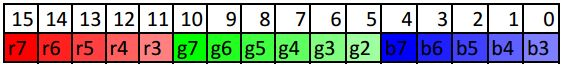##### converting RGB888 into RGB565 in C/C++

Let’s assume we have 3 individual input bytes, one for each color. I use

`uint8_t`

to represent a byte but

`char`

and others do the same. The code for converting a 4 byte type such as

`int //check your compiler if int is 4 byte type`

or

`uint32_t`

can be found after this section.

```uint8_t red;
uint8_t green;
uint8_t blue;```

The result shall be a

`uint16_t`

(16 bit/2 byte)

`uint16_t Rgb565 = 0;`

Assigning a 0 to

`Rgb565`

will result in all bits being 0.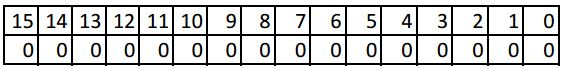In a first step, we have to shift in the upper 5 bits of

`red`

. Why the upper bits? Because they have the highest significance/impact on the color. To get ONLY the upper 5 bits, we need to mask them. Masking is done by a bitwise AND operation (

`&`

); Only if a bit is 1 in both numbers, the result is 1, otherwise 0. This is  the point where we loose information, as we mask out fine shades of red.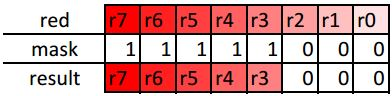The bit pattern 11111000 can be represented by

`0b11111000`

in binary;

`0xf8`

in hex; or

`248`

in decimal. Which formats are available depends on your compiler, I will go with binary as I think its most visual.

After we have masked the 5 upper bits, we need to shift

`red`

by 8 bits to the left.

`Rgb565 = (red & 0b11111000) <<8 ;`

`red`

by 8 to the left. The result is: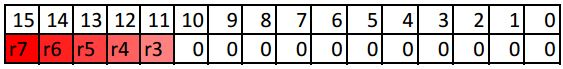Now we add the green portion, here we have a mask of 6 bits.

`Rgb565 = Rgb565 + ((green & 0b11111100 ) <<3);`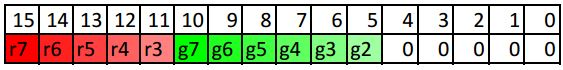`+`

) the new bits works, because the unused bits are all 0. If they would have other values, adding wouldn’t work as the resulting carry bits (of the addition) would corrupt the result.

Finally we add blue, which is shifted to the right. Masking can be skipped here, since the unused bits are shifted out of the range of

`Rgb565`

.

`Rgb565 = Rgb565 + ((blue) >> 3);`Of course, the whole conversion can be put in a single line:

`Rgb565 = (((red & 0b11111000)<<8) + ((green & 0b11111100)<<3)+(blue>>3))`

Or with hex format

`Rgb565 = (((red & 0xf8)<<8) + ((green & 0xfc)<<3)+(blue>>3))`

##### 4 byte (int/uint32_t) input

If the input is a 4 byte type such as

`int //check your compiler if int is 4 byte type`

or

`uint32_t`

, the principle of masking and shifting is the same, though we always refer to the same input variable instead of individual ones.

Lets assume we have

`int RGB888;`

as input- and

`uint16_t RGB565;`

as output- variable.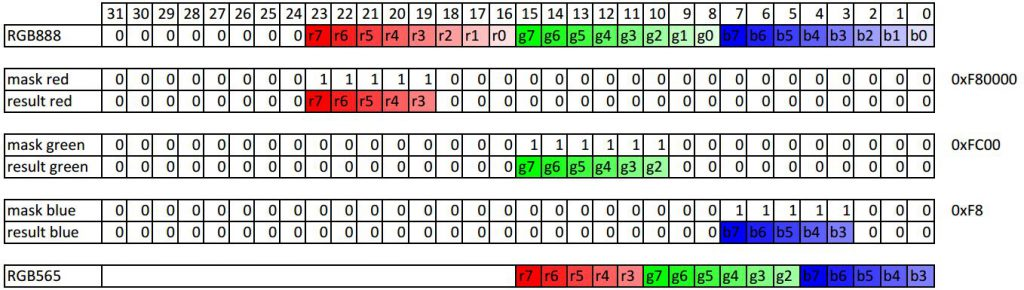Hence, the conversion looks like this:

`RGB565 = (((RGB888&0xf80000)>>8) + ((RGB888&0xfc00)>>5) + ((RGB888&0xf8)>>3));`

### 1 thought on “RGB565 Color Picker”

1. Thanx for this great web tool. Very useful. And your website looks very interesting. Best regards, DOMin8or

This site uses cookies. By continuing to browse the site you are agreeing to our use of cookies.